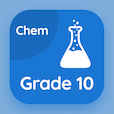Engineering Online Courses

Engineering Physics Quizzes

Engineering Physics Quiz PDF - Complete

# Period of Rotation of Planets Multiple Choice Questions p. 104

Study Period of Rotation of Planets multiple choice questions and answers, period of rotation of planets quiz answers PDF 104 to study Engineering Physics course online. Astronomical Data MCQ trivia questions, Period of Rotation of Planets Multiple Choice Questions (MCQ) for online college degrees. "Period of Rotation of Planets MCQ" PDF eBook: vectors and scalars, planet and satellites: kepler law, reflection and refraction, angular simple harmonic oscillator, period of rotation of planets test prep for online engineering graduate schools.

"Period of rotation of Venus w.r.t distant stars is" MCQ PDF: −-0.451 d, 0.997 d, −243 d, and 0.897 d for engineering graduate schools. Learn astronomical data questions and answers to improve problem solving skills for college entrance exams.

## Period of Rotation of Planets Questions and Answers MCQs

MCQ: Period of rotation of Venus w.r.t distant stars is

0.997 d
−-0.451 d
−243 d
0.897 d

MCQ: A pendulum that rotates rather then swing is called

Torison pendulum
Tension pendulum
Spring pendulum
Equilibrium pendulum

MCQ: Phenomena in which change in direction of a particular wave when it bounce off a barrier is called

reflection
refraction
retardation
attenuation

MCQ: If p is the semi-latus rectum and ε is eccentricity, then Ellipse can be represented by formula

P/(1+εcosθ)
P/(1+εsinθ)
P/(1+εtanθ)
P/(1+εcotθ)

MCQ: Velocity is a

vector quantity
unit vector
scalar quantity
infinite quantity

### More Quizzes from Engineering Physics Course